# Fixed Probes

There are many types of fixed probe as described in the following table

These probes are simply schematic symbols with special properties. When you place a fixed probe on the schematic, the probed value at the point where you place the probe will be plotted each time you run the simulation.

Current probes and power probes must be placed directly over a part pin. They will have no function if they are not and a warning message will be displayed.

In this topic:

## Fixed Probe Options

All probe types have a large number of options allowing you to customise how you want the graph plotted. For many applications the default settings are satisfactory. In this section, the full details of available probe options are described. Select the probe and press F7 or menu Edit Part... The following dialog will be displayed for voltage, current, power, db and phase probes: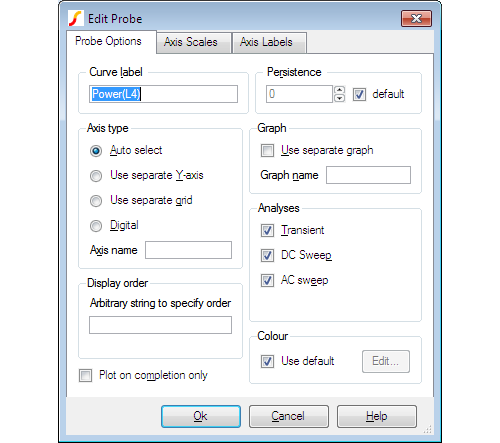The elements of each tabbed sheet are explained below.

### Probe Options Sheet

Curve Label Text displayed by the probe on the schematic and also used to label the resulting curves
Persistence The number of curves from a single probe that will be displayed at once.
• If non-zero, curves created from the curve will have a limited lifetime with the oldest being automatically deleted first.
• If set to zero, the curves will never be deleted.
If default is checked, the number of curves will be based on the values specified in the general options.

To set the persistence value in general options, follow these steps:
1. From the menu bar, select File > Options > General..., and then select the Graph/Probe/Data Analysis tab
2. In the Fixed probe global options section, enter a value in the Default persistence field, and then click Ok

Axis type
Specifies the type of y-axis to use for the curve.
 Auto Select Uses the main y-axis unless its units are incompatible; for example, if you plot a current on a graph that already has a voltage, a new y-axis will be created alongside the main one. See diagram in section Graph Layout - Multiple Y-Axis Graphs. If the signal is digital, a digital axis (see below) will be used for this probe. Use Separate Y-axis Uses its own separate y-axis. If you specify this you can optionally supply an axis name that is arbitrary and used to identify the axis so that multiple fixed probes can specify the same one. This name is not used as a label for display purposes but simply as a means of identification. Axes can be labelled using the Axis Labels sheet as explained below. Use Separate Grid Similar to above but uses a new grid that is stacked on top of main grid. See diagram in section Graph Layout - Multiple Y-Axis Graphs. Digital Use a digital axis. Digital axes are placed at the top of the window and are stacked. Each one may take only a single curve. As the name suggests, these are intended for digital traces but can be used for analog signals if required.

Graph
Check Use Separate Graph to create a new graph sheet for the probe. You may also supply a graph name, which works the same way as axis name described above. this is not a label but a means of identification. Any other probes using the same graph name will have their curves directed to the same graph sheet.

Analyses
Specifies for which analyses the probe is enabled.

Note: Other analysis modes such as noise and sensitivity are not included because these do not support schematic cross probing of current or voltage. For SIMPLIS mode, a Periodic Operating Point (POP) option is also available.

Display order
Enter a string to control the grid display order.

The value is arbitrary and will not be displayed. To force the curve to be placed above other curves that don't use this value, prefix the name with '!'. The '!' character has a low ASCII value. Conversely, use '~' to force curve to be displayed after other curves.

Colour
Check Use default to minimise duplicate colours on the same graph by allowing the colour to be chosen automatically. Alternatively uncheck, this box and then press Edit... to select a colour of your choice. In this case the trace always has the same colour.

Plot on completion only

• If checked, incremental plotting is disabled; that is, the plot does not appear until the run is complete.
• If not checked, the curve updates regularly during the course of the run.
Note: For some expressions, it is not possible to incrementally plot the data; if this is the case incremental plotting is disabled and this check box is ignored.

### Axis Scales Sheet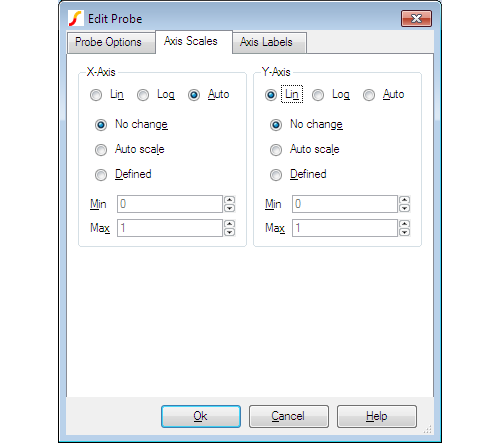Parameter Description Lin/Log/Auto Specify whether you want X-Axis to be linear or logarithmic. If you select Auto, the axis (X or Y) will be set to log if the x values are logarithmically spaced. For the Y-axis, it is also necessary that the curve values are positive for a log axis to be selected. No Change Auto scale Defined Controls how the axis limits are defined If you select No change, the axis scales remain as they are; this is relevant only if you are adding to an existing graph. If you select Auto scale, the axis scales are based on the minimum and maximum values of the curve. If you select Defined, enter values in the Min and Max fields.

### Axis Labels Sheet

This sheet has four edit boxes allowing you to specify, x and y axis labels as well as their units. If any box is left blank, a default value will be used or will remain unchanged if the axis already has a defined label.

## Fixed Bus Probe Options

Select device and press F7 in the usual manner. A dialog box will show similar to that shown in Bus Probe Options. But you will notice an additional tabbed sheet titled Probe Options. This allows you to select an axis type and graph in a similar manner to that described above for fixed voltage and current probes.

## Using Fixed Probes in Hierarchical Designs

Fixed probes may successfully be used in hierarchical designs. If placed in a child schematic, a plot will be produced for all instances of that child and the labels for each curve will be prefixed with the child reference.

## Adding Fixed Probes After a Run has Started

When you add a fixed single ended voltage or current probe after a run has started, the graph of the probed point opens soon after resuming the simulation. To do this:

1. Pause simulation.
2. Place a probe on the circuit in the normal way.
3. Resume simulation

## Per-cycle Probes

Per Cycle Probes first calculate a new curve based on a built-in formula and then plot the curve on a graph. The input can be one of the following:
• Single-ended voltage
• Differential voltage
• Current
• Difference of two currents
As with the other probes, the curve can be output to any graph and axis. Per Cycle Probes are compatible with both simulators.
The Per-cycle Probe specification is shown in the following table:
 Per-cycle Probes Model Name: Per Cycle Probes Simulator: The device is compatible with the SIMetrix and SIMPLIS simulators SIMetrix Parts Selector Location: Probes > Per Cycle Voltage Probes Probes > Per Cycle Diff Voltage Probes Probes > Per Cycle Current Probes Probes > Per Cycle Diff Current Probes SIMPLIS Parts Selector Location: Commonly Used Parts > Per Cycle Voltage Probes Commonly Used Parts > Per Cycle Diff Voltage Probes Commonly Used Parts > Per Cycle Current Probes Commonly Used Parts > Per Cycle Diff Current Probes Symbol Library: None the symbol is automatically generated when placed Symbols: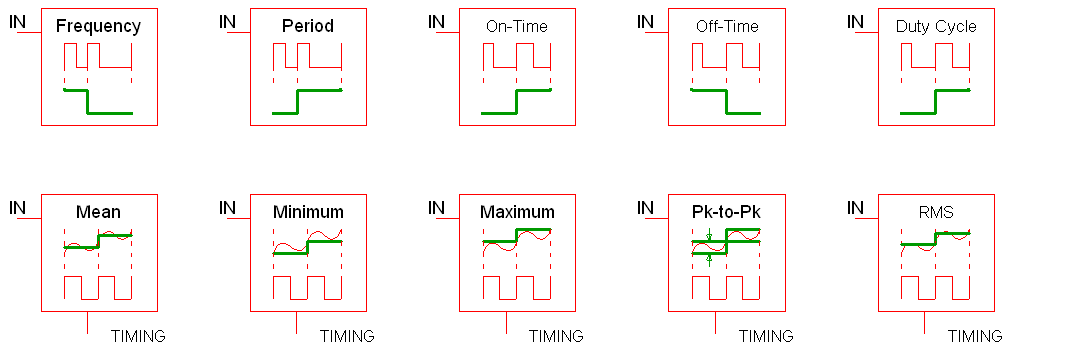Multiple Selections: Multiple probes can be selected and edited simultaneously.

### Per-Cycle Timing Measurements

These advanced probes allow you to visualize subtle behaviour which is not otherwise easy to see. One example is to visualize how the switching frequency varies over the simulation time window. In this case, the probe first finds all edges which match the trigger conditions and then calculates a new curve with the frequency measurements on the vertical axis and the input time vector on the horizontal axis. An example waveform showing a pulse train and the per cycle frequency of the curve is shown below: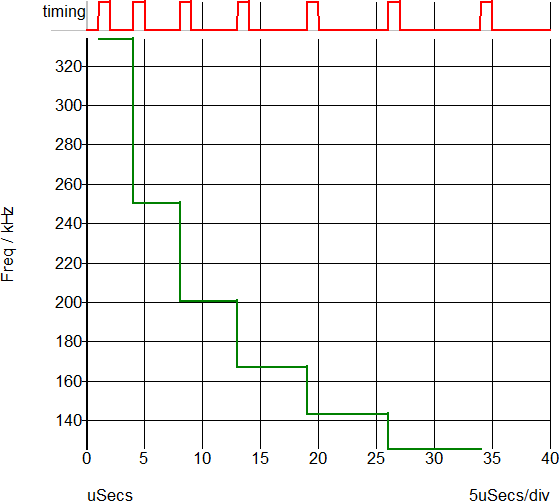The following timing measurements are supported on a per-cycle basis:
• Frequency
• Period
• Duty Cycle
• On Time
• Off Time

### Per-Cycle Amplitude Measurements

The per-cycle measurement can also be applied to amplitude of an input voltage or current. For example, you can plot the mean value of a switching power converter output with the mean value being calculated on a per-cycle basis. This example is show below - the mean value of a converter output voltage is plotted in a per-cycle manner. The gate voltage is used to determine the timing edge information.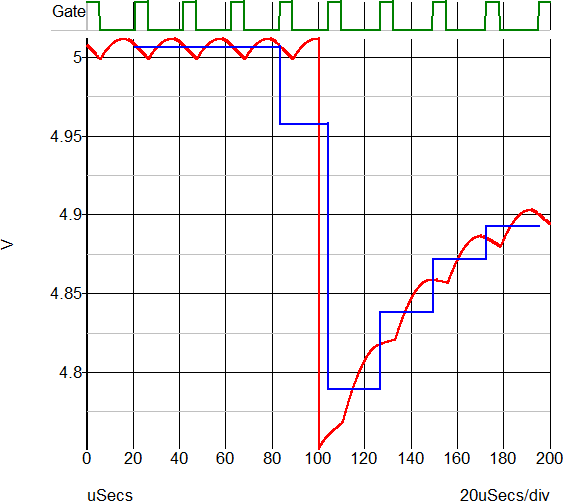The following amplitude measurements are supported:
• Mean
• Maximum
• Minimum
• RMS
• Peak to Peak

### Editing the Per Cycle Probe

To configure the Per Cycle Probe, follow these steps:
1. Double click the symbol on the schematic to open the editing dialog to the Probe Options tab.
2. Make the appropriate changes on the three tabs as explained below.

### Per Cycle Probe Options

The following tab allows you to define general probe options, which are explained in the table below.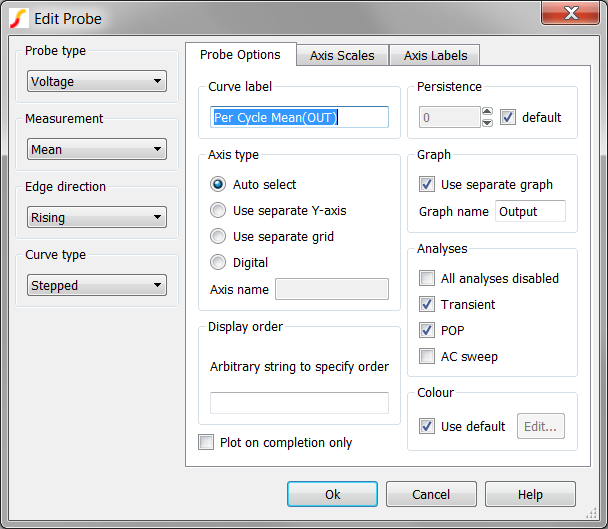Parameter Description
Measurement Frequency, Period, On-Time, Off-Time, Duty Cycle, Mean Maximum, Minimum, Peak-to-Peak, or RMS

Edge

Direction
Rising, Falling or Both
Curve type Stepped or Smooth
Curve Label Text displayed by the probe on the schematic and also used to label the resulting curves
Persistence The number of curves from a single probe that will be displayed at once.
• If non-zero, curves created from the curve will have a limited lifetime with the oldest being automatically deleted first.
• If set to zero, the curves will never be deleted.
If default is checked, the number of curves will be based on the values specified in the general options.

To set the persistence value in general options, follow these steps:
1. From the menu bar, select File > Options > General..., and then select the Graph/Probe/Data Analysis tab
2. In the Fixed probe global options section, enter a value in the Default persistence field, and then click Ok

Axis type
Specifies the type of y-axis to use for the curve.
 Auto Select Uses the main y-axis unless its units are incompatible; for example, if you plot a current on a graph that already has a voltage, a new y-axis will be created alongside the main one. See diagram in section Graph Layout - Multiple Y-Axis Graphs. If the signal is digital, a digital axis (see below) will be used for this probe. Use Separate Y-axis Uses its own separate y-axis. If you specify this you can optionally supply an axis name that is arbitrary and used to identify the axis so that multiple fixed probes can specify the same one. This name is not used as a label for display purposes but simply as a means of identification. Axes can be labelled using the Axis Labels sheet as explained below. Use Separate Grid Similar to above but uses a new grid that is stacked on top of main grid. See diagram in section Graph Layout - Multiple Y-Axis Graphs. Digital Use a digital axis. Digital axes are placed at the top of the window and are stacked. Each one may take only a single curve. As the name suggests, these are intended for digital traces but can be used for analog signals if required.

Graph
Check Use Separate Graph to create a new graph sheet for the probe. You may also supply a graph name, which works the same way as axis name described above. this is not a label but a means of identification. Any other probes using the same graph name will have their curves directed to the same graph sheet.

Analyses
Specifies for which analyses the probe is enabled.

Note: Other analysis modes such as noise and sensitivity are not included because these do not support schematic cross probing of current or voltage. For SIMPLIS mode, a Periodic Operating Point (POP) option is also available.

Display order
Enter a string to control the grid display order.

The value is arbitrary and will not be displayed. To force the curve to be placed above other curves that don’t use this value, prefix the name with '!'. The '!' character has a low ASCII value. Conversely, use '~' to force curve to be displayed after other curves.

Colour
Check Use default to minimise duplicate colours on the same graph by allowing the colour to be chosen automatically. Alternatively uncheck, this box and then press Edit... to select a colour of your choice. In this case the trace always has the same colour.

Plot on completion only
This option is not available with per-cycle probes and the setting of the check box will be ignored.

### Axis Scales

The following tab allows you to define the scale for the X-axis and for the Y-axis as explained in the table below.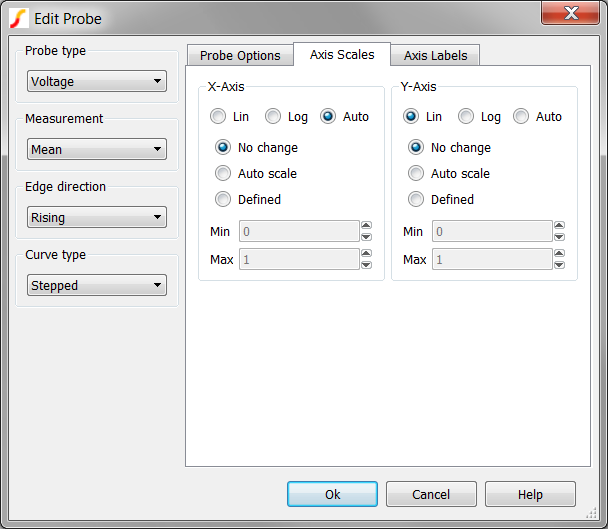Parameter Description Lin/Log/Auto Specify whether you want X-Axis to be linear or logarithmic. If you select Auto, the axis (X or Y) will be set to log if the x values are logarithmically spaced. For the Y-axis, it is also necessary that the curve values are positive for a log axis to be selected. No Change Auto scale Defined Controls how the axis limits are defined If you select No change, the axis scales remain as they are; this is relevant only if you are adding to an existing graph. If you select Auto scale, the axis scales are based on the minimum and maximum values of the curve. If you select Defined, enter values in the Min and Max fields.

### Axis Labels

To specify axis labels and units, click the Axis Labels tab, and enter values as needed.

Note: If any box is left blank, a default value is used or remains unchanged if the axis already has a defined label.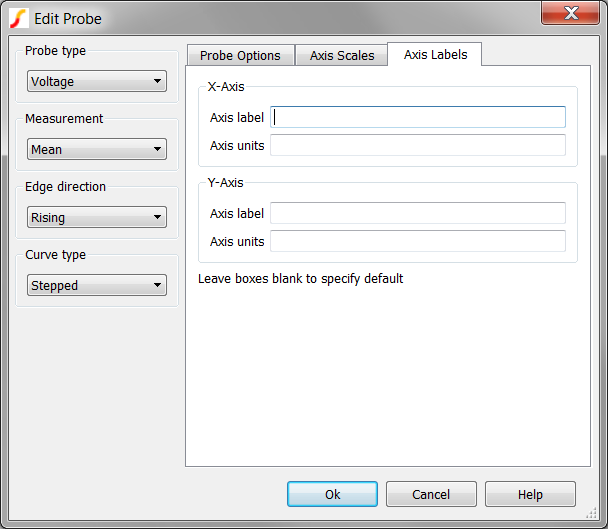## XY Probes

XY Probes plot two curves against each other in an XY plot. Each input can be either differential voltage or inline current, and the curves can be output to any graph and axis. XY probes are available in both SIMetrix and SIMPLIS. The XY Probe specification is shown in the following table:
 XY Probes Model Name: XY Probe Simulator: This device is compatible with the SIMetrix and SIMPLIS simulators. Part Selector Location: Probes > XY Probes Symbol Library: connection.sxslb Symbols: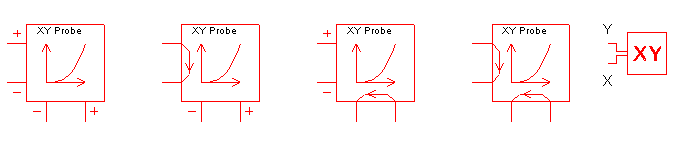Multiple Selections: Multiple probes can be selected and edited simultaneously.

### Editing the XY Probe

To configure the XY Probe, follow these steps:
1. Double click the symbol on the schematic to open the editing dialog to the Probe Options tab.
2. Make the appropriate changes on the three tabs as explained below.

### XY Probe Options

The following tab allows you to define general probe options, which are explained in the table below.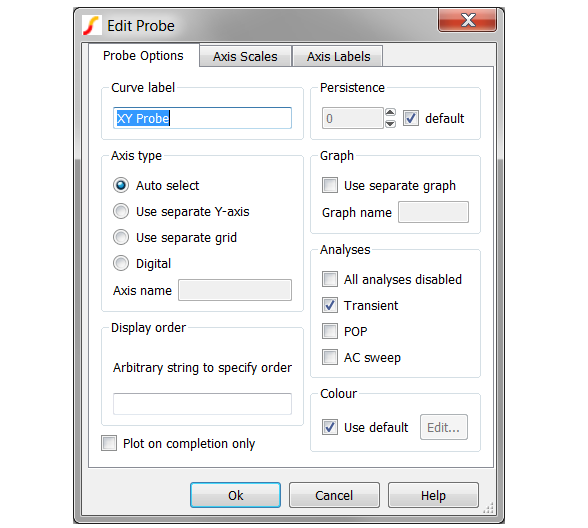Curve Label Text displayed by the probe on the schematic and also used to label the resulting curves
Persistence The number of curves from a single probe that will be displayed at once.
• If non-zero, curves created from the curve will have a limited lifetime with the oldest being automatically deleted first.
• If set to zero, the curves will never be deleted.
If default is checked, the number of curves will be based on the values specified in the general options.

To set the persistence value in general options, follow these steps:
1. From the menu bar, select File > Options > General..., and then select the Graph/Probe/Data Analysis tab
2. In the Fixed probe global options section, enter a value in the Default persistence field, and then click Ok

Axis type
Specifies the type of y-axis to use for the curve.
 Auto Select Uses the main y-axis unless its units are incompatible; for example, if you plot a current on a graph that already has a voltage, a new y-axis will be created alongside the main one. See diagram in section Graph Layout - Multiple Y-Axis Graphs. If the signal is digital, a digital axis (see below) will be used for this probe. Use Separate Y-axis Uses its own separate y-axis. If you specify this you can optionally supply an axis name that is arbitrary and used to identify the axis so that multiple fixed probes can specify the same one. This name is not used as a label for display purposes but simply as a means of identification. Axes can be labelled using the Axis Labels sheet as explained below. Use Separate Grid Similar to above but uses a new grid that is stacked on top of main grid. See diagram in section Graph Layout - Multiple Y-Axis Graphs. Digital Use a digital axis. Digital axes are placed at the top of the window and are stacked. Each one may take only a single curve. As the name suggests, these are intended for digital traces but can be used for analog signals if required.

Graph
Check Use Separate Graph to create a new graph sheet for the probe. You may also supply a graph name, which works the same way as axis name described above. this is not a label but a means of identification. Any other probes using the same graph name will have their curves directed to the same graph sheet.

Analyses
Specifies for which analyses the probe is enabled.

Note: Other analysis modes such as noise and sensitivity are not included because these do not support schematic cross probing of current or voltage. For SIMPLIS mode, a Periodic Operating Point (POP) option is also available.

Display order
Enter a string to control the grid display order.

The value is arbitrary and will not be displayed. To force the curve to be placed above other curves that don’t use this value, prefix the name with '!'. The '!' character has a low ASCII value. Conversely, use '~' to force curve to be displayed after other curves.

Colour
Check Use default to minimise duplicate colours on the same graph by allowing the colour to be chosen automatically. Alternatively uncheck, this box and then press Edit... to select a colour of your choice. In this case the trace always has the same colour.

Plot on completion only
This option is not available with XY Probes and the check box setting will be ignored.

### Axis ScalesParameter Description Lin/Log/Auto Specify whether you want X-Axis to be linear or logarithmic. If you select Auto, the axis (X or Y) will be set to log if the x values are logarithmically spaced. For the Y-axis, it is also necessary that the curve values are positive for a log axis to be selected. No Change Auto scale Defined Controls how the axis limits are defined If you select No change, the axis scales remain as they are; this is relevant only if you are adding to an existing graph. If you select Auto scale, the axis scales are based on the minimum and maximum values of the curve. If you select Defined, enter values in the Min and Max fields.

### Axis Labels

To specify axis labels and units, click the Axis Labels tab, and enter values as needed.

Note: If any box is left blank, a default value is used or remains unchanged if the axis already has a defined label.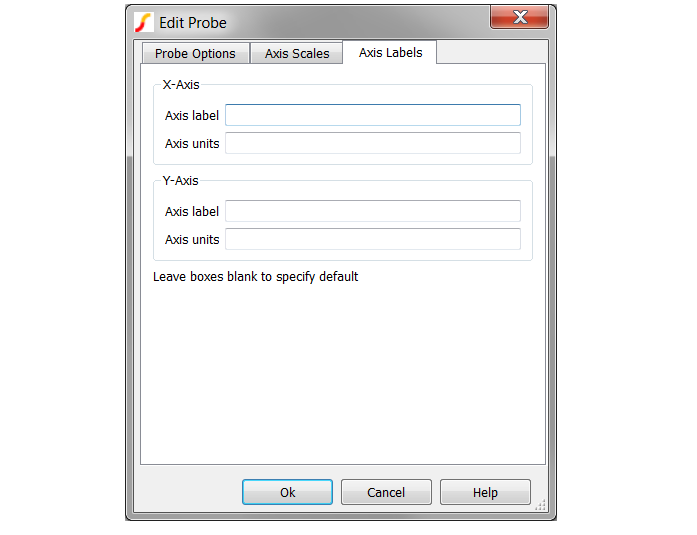## Bode Plot Probe with Measurements

The Bode Plot Probe with Measurements generates plots for the gain and phase of the ratio of two voltages. The probe can be configured to plot only the gain or phase, or both gain and phase.

 Summary - Bode Plot Probe with Measurements Model Name Bode Plot Probe Simulator This device is compatible with both SIMetrix and SIMPLIS simulators Schematic Menu Probe AC/Noise > Bode Plot Probe - with Measurements Parts Selector Probes > Bode Plot Probe - with Measurements Symbol Library Connections Model File none Symbol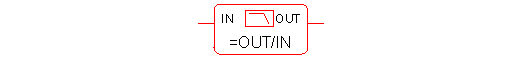Multiple Selections If multiple Bode plot probes are selected before editing, all properties except the curve labels can be simultaneously changed for all probes. The curve label properties will remain unchanged for all selected probes Usage This schematic probe symbol plots the magnitude and phase of the ratio of two complex voltages, OUT/IN. The magnitude can be plotted in db or volts/volts; the horizontal axis scale is determined by the simulation sweep type. If the simulation is swept in log frequency steps, the horizontal axis will automatically be log scaled

### Editing the Bode Plot Probe with Measurements

To configure the Bode Plot Probe with Measurements, follow these steps:
1. Double click the symbol on the schematic to open the editing dialog to the Parameters tab.
2. Make the appropriate changes to the fields described in the table below the image.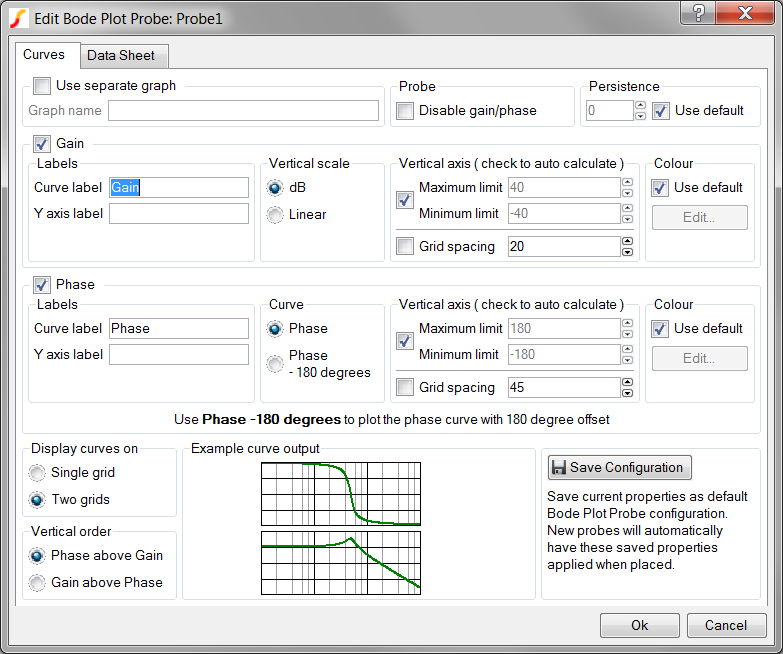Options - Bode Plot Probe with Measurements Use separate graph Check this option in order to supply an alias for each output graph tab Graph name The Graph name is an alias for each output graph tab and will not be visible on the graph output. Curves with the same graph name property will be output on the same graph tab. Graph name be safely ignored unless multiple Bode plot probes are used. Use separate graph must be checked to supply this name. Disable gain/phase Check this box to disable both gain and phase graphs Persistence The Persistence property determines when previous simulation data is deleted from the graph viewer. If Use default is checked or if the persistence is set to -1, the probe uses the default global persistence value. The default persistence can be set from the command shell menu: File > Options > General If set to "0", the graph data is never deleted, and subsequent simulations will add new curves to the same axis locations If set to a positive integer, the persistence value will be interpreted as the number of simulations to keep on the graph. For example, a persistence setting equal to 1 keeps only the current data, and each subsequent simulation overwrites the graph. A setting equal to 2 keeps the current data and the data from the previous simulation Curve label Sets the name of the curve. Note: This field appears in both the Gain and Phase groups on the dialog Y axis label Sets the Y axis label for the individual gain and phase axes. When multiple curves from multiple probes are placed on the same axis, the axis label properties must be identical or the axis label is blank. If this field is left blank, the axis label appears with the name specified in the Curve label field. Note: This field appears in both the Gain and Phase groups on the dialog Vertical scale Allows you to select the function to perform on the simulation data. Select db to perform the built-in db() vector function. Select Linear to perform the mag() function Curve Selects the Phase curve offset Select Phase for no offset. Select Phase - 180 degrees to subtract 180 degrees before plotting the curve. Vertical axis This group has two options: Vertical limits Check to have the vertical limits automatically set to fit the curve data. Uncheck to enter minimum and maxium values for the limits. Both minimum and maximum axis limits must be set or auto-calculated as a group. Grid spacing: Check to auto calculate the grid spacing. Uncheck to set the grid spacing independent of the axis limits Note: This field appears in both the Gain and Phase groups on the dialog. Colour Defines colours for the curves: Check Use default in both the Gain and Phase groups o generate the curves with the same default colour, which is automatically selected from a sequence of colours Leave Use default unchecked and click Edit... to open a colour selector dialog box to specify fixed colours for the curves The change the default sequence of colours from the command shell menu, select File > Options > Colour For additional information see Colours and Fonts. Note: This field appears in both the Gain and Phase groups on the dialog. Display curves on Allows you to define the number of grids in the output: Single grid or Two grids Vertical order Allows you to specify the order of the curves: Phase above Gain or Gain above Phase. If you selected Two grids is in the Display curves on group, the selection in this group specifies the curve output location If you selected Single grid, both curves are on the lower grid with two axes Example curve output Example curve output illustrates the relative locations of the two curves. Note: The curve data here is fixed; these curves are examples and do not reflect the simulated curves Save Configuration Click Save Configuration to preserve this information as the default configuration for all future new Bode plot probes

## Bode Plot Probe - Basic Version

Connects to the input and output of a circuit to plot its gain and phase. A more sophisticated bode plot probe which provides a wide range of options along with the display of useful measurements is also available and is recommended for most applications. See Bode Plot Probe with Measurements

This basic version is useful for quick checks and for compatibility with versions 7.0 or earlier.

 Summary - Bode Plot Probe - Basic Model Name Bode Plot Probe - Basic Simulator This device is compatible with both SIMetrix and SIMPLIS simulators Schematic Menu Probe AC/Noise > Bode Plot Probe - Basic Parts Selector Probes > Bode Plot - Gain/Phase Symbol Library Connections Model File none Symbol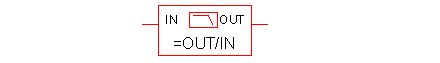Multiple Selections If multiple Bode plot probes are selected before editing, all properties except the curve labels can be simultaneously changed for all probes. The curve label properties will remain unchanged for all selected probes Usage This schematic probe symbol plots the magnitude and phase of the ratio of two complex voltages, OUT/IN. The magnitude can be plotted in db or volts/volts; the horizontal axis scale is determined by the simulation sweep type. If the simulation is swept in log frequency steps, the horizontal axis will automatically be log scaled

### Editing Bode Plot Probe

To configure the Bode Plot Probe, follow these steps:
1. Double click the symbol on the schematic to open the editing dialog to the Parameters tab.
2. Make the appropriate changes to the fields described in the table below the image.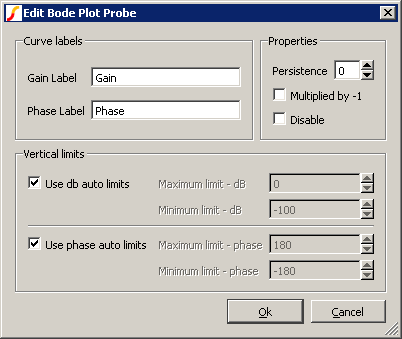Options - Bode Plot Probe Curve labels Sets labels for plots Gain label: Label used for gain plot Phase label: Label used for phase plot Properties Set additional properties on probe: Persistence: Controls how many plots are retained. 0 means use global default setting Multiplied by -1: Inverts the sense of the phase Disable: Disable probe. If checked no plots will be created Vertical Limits Divided into two parts to allow setting of axis limits for phase and gain plots. Use db auto limits: If checked the gain plot will automatically scale. Otherwise manual limits can be entered in Maximum limit - dB and Minimum limit - dB Use phase auto limits: If checked the phase plot will automatically scale. Otherwise manual limits can be entered in Maximum limit - phase and Minimum limit - phase

## Fourier Analysis

A fixed Fourier probe is available which will perform a spectral analysis on a node voltage. To place this probe, select menu Probes > Place Fourier Voltage Probe.

Double click the probe to edit it. You will see this dialog box: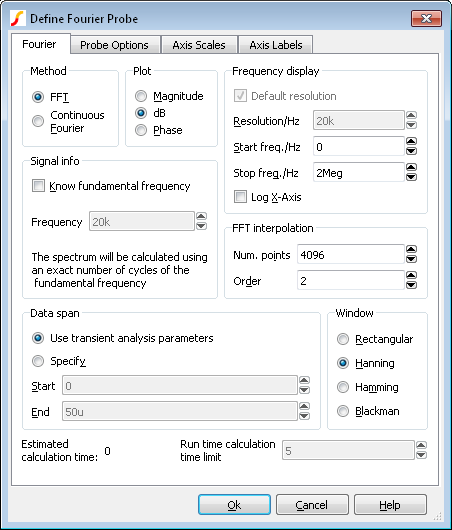The settings are similar to that for random Fourier probe plotting as described here: Fourier Analysis. The documentation is repeated here for convenience.

### Method

SIMetrix offers two alternative methods to calculate the Fourier spectrum: FFT and Continuous Fourier.

The simple rule is: use FFT unless the signal being examined has very large high frequency components as would be the case for narrow sharp pulses.

A description of the two techniques and their pros and cons follows.

 FFT Fast Fourier Transform. This is an efficient algorithm for calculating a discrete Fourier transform or DFT. DFTs generally operate on evenly spaced sampled data. Unfortunately the data generated by the simulator is not evenly spaced so it is therefore necessary to interpolate the data before presenting it to an FFT algorithm. The interpolation process is in effect the sampling process and the Nyquist sampling theorem applies. This states that the signal can be perfectly reproduced from the sampled data if the sampling rate is greater than twice the maximum frequency component in the signal. In practice this condition can never be met perfectly and any signal components whose frequency is greater than half the sampling rate will be aliased to a different frequency. So if the number of interpolated points is too small there will be errors in the result due to high frequency components being aliased to lower frequencies. This is the Achilles heel of FFTs applied to simulated data. The Continuous Fourier technique, described next, does not suffer from this problem. It suffers from other problems the main one being that it is considerably slower than the FFT. Continuous Fourier This calculates the Fourier spectrum by numerically integrating the Fourier integral. With this method, each frequency component is calculated individually whereas with the FFT the whole spectrum is calculated in one - quite efficient - operation. Continuous Fourier does not require the data to be interpolated and does not suffer from aliasing. The problem with continuous Fourier is that compared to the FFT it is a slow algorithm and in many cases an FFT with a very large number of interpolated points can be calculated more quickly and give just as accurate a result. However, in cases where a signal has a very large high frequency content - such as narrow pulses - this method is superior and it is recommended that it is used in preference to the FFT in such situations. The continuous Fourier technique has the additional advantage that it can be applied with greater confidence as the aliasing errors will not be present. It does have its own source of error due to the fact that simulated data itself is not truly continuous but represented by unevenly spaced points with no information about what lies between the points. This error can be minimised by ensuring that close simulation tolerances are used. See Simulator Reference Manual/Convergence, Accuracy and Performance for details.

### Plot (Phase or Magnitude)

The default is to plot the magnitude of the Fourier spectrum. Select Phase if you require a plot of phase or dB if you need the magnitude in dBs.

### Frequency Display

 Resolution/Hz Available only for the continuous Fourier method. This is the frequency interval at which the spectral components are evaluated. It cannot be less than 1/T where T is the time interval over which the spectrum is calculated. Start Freq./Hz Start frequency of the display. Stop Freq./Hz Stop frequency of the display. Log X-Axis Check this to specify a logarithmic x-axis. This will force a minimum value for the start frequency equal to 1/T where T is the time interval being analysed.

### Signal Info

If the signal being analysed is repetitive and the frequency of that signal is known exactly then a much better result can be obtained if it is specified here. Check the Know fundamental frequency box then enter the frequency. The Fourier spectrum will be calculated using an integral number of complete cycles of the fundamental frequency. This substantially reduces spectral leakage. Spectral leakage occurs because both the Fourier algorithms work on an assumption that the signal being analysed is a repetition of the analysed time interval from $t=-\infty$ to $t=+\infty$. If the analysed time interval does not contain a whole number of cycles of the fundamental frequency this will be a poor approximation and the spectrum will be in error. In practice this problem is minimised by using a window function applied to the signal prior to the Fourier calculation, but using a whole number of cycles reduces the problem further.

Note that the fundamental frequency is not necessarily the lowest frequency in the circuit but the largest frequency for which all frequencies in the circuit are integral harmonics. For example if you had two sine wave generators of 1kHz and 1.1KHz, the fundamental is 100Hz, not 1kHz; 1kHz is the tenth harmonic, 1.1KHz is the eleventh.

You should not specify a fundamental frequency for circuits that have self-oscillating elements.

### FFT Interpolation

As explained above, the FFT method must interpolate the signal prior to the FFT computation. Specify here the number of points and the order. The number of points entry may be forced to a minimum if a high stop frequency is specified in the Frequency Display section.

The number of interpolation points required depends on the highest significant frequency component in the signal being analysed. If you have an idea what this is, a useful trick to set the number of points to a suitable value, is to increase the stop frequency value in the Frequency Display section up to that frequency. This will automatically set the number of interpolation points to the required value to handle that frequency. If you don't actually want to display frequencies up to that level, you can bring the stop frequency back down again. The number of interpolation points will stay at the value reached.

If in doubt, plot the FFT twice using a different number of points. If the two results are significantly different in the frequency band of interest, then you should increase the number of points further.

Usually an interpolation order of 2 is a suitable value but you should reduce this to 1 if analysing signals with abrupt edges. If analysing a smooth signal such as a sinusoid, useful improvements can be gained by increasing the order to 3.

### Data Span

Usually the entire simulated time span is used for the Fourier analysis. To specify a smaller time interval click Specify and enter the start and end times.

Note that if you specify a fundamental frequency, the time may be modified so that a whole number of cycles is used. This will occur whether or not you explicitly specify an interval.

### Window

A window function is applied to the time domain signal to minimise spectral leakage (See above).

The choice of window is a compromise. The trade off is between the bandwidth of the main spectral component or lobe and the amplitude of the side-lobes. The rectangular window - which is in effect no window - has the narrowest main lobe but substantial side-lobes. The Blackman window has the widest main lobe and the smallest side lobes. Hanning and Hamming are something in between and have similar main lobe widths but the side lobes differ in the way they fall away further from the main lobe. Hamming starts smaller but doesn't decay whereas Hanning while starting off larger than Hamming, decays as the frequency moves away from the central lobe.

Despite the great deal of research that has been completed on window functions, for many applications the difference between Hanning, Hamming and Blackman is not important and usually Hanning is a good compromise.

There are situations where a rectangular window can give significantly superior results. This requires that the fundamental frequency is specified and also that the simulated signal is consistent over a large number of cycles. The rectangular window, however, usually gives considerably poorer results and must be used with caution.

### Run time calculation time limit

If using the Continuous Fourier method, you can enter a maximum time limit to calculate the Fourier spectrum. If the limit is exceeded, the calculation will abort and no display will be made. The Continuous Fourier calculation time can be excessive but cannot be predicted before the simulation is started as it is necessary to know the number of simulation time points to be processed.

For the FFT method, the simulation time can be estimated to a reasonable accuracy before the simulation and this estimate will be displayed labelled Estimated calculation time:.

## Custom Fixed Probe

You can create an arbitrary fixed probe to plot an expression of any combination of node voltages and device currents. To use this feature, select menu Probe > Create and Place Arbitary Probe. You will see this dialog box: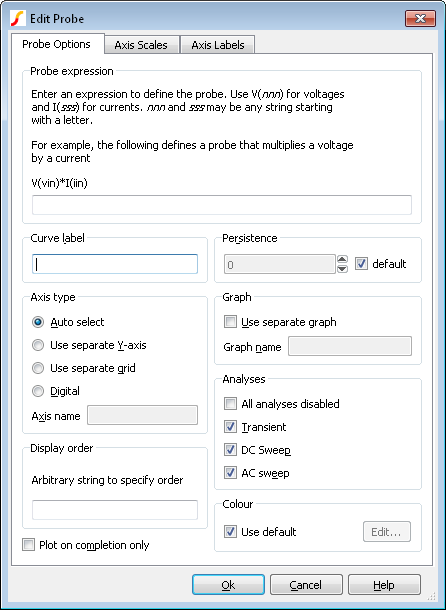### Probe expression

Enter an expression to define what you wish to be plotted. Use V(nnn) to access a voltage and I(sss) to access a current. nnn and sss may be any arbitrary string starting with a letter. When you close the dialog box a symbol will be created which will reflect what you enter here. It will have single inputs named according to occurrences of V(nnn) and pairs of inputs named after occurrences of I(sss). For example if you enter the expression:

V(vin)*I(iin)

The symbol created will look like this:

\begin{figure}[H] \centering \includegraphics[width=0.4\linewidth]{images/Custom-probe-symbol.png} \end{figure}

The result plotted will be the product of the voltage on vin and the current in iin.

The same behaviour could be achieved using a Non-linear transfer function device (see Non-linear Transfer Function ) and a simple single ended probe. This arbitrary probe has some important advantages over that approach:

1. The arbitrary probe works in SIMPLIS simulations. The Non-linear transfer function device is a SIMetrix-only device.
2. It can evaluate non-linear functions in AC analysis
3. It is a post-processing operation and does not interfere with the simulation in any way

### Other Arbitrary Probe Options

The other options are the same as for standard fixed probes. Refer to Fixed Probe Options

## Changing Update Period and Start Delay

The update period of all fixed probes can be changed from the Options dialog box. Select menu File > Options > General... and click on the Graph/Probe/Data analysis tab. In the Probe update times/seconds box there are two values that can be edited. Period is the update period and Start is the delay after the simulation begins before the curves are first created.